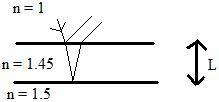# Interference from Thin Films

• baseballer10p

## Homework Statement

Light of wavelength x, in air, is incident in air on a film of oil with index of refraction n_oil = 1.45. The film of oil has thickness L, and is spread on glass with an index of refraction n_glass = 1.50.
The wavelength of the light in oil will be _____ than the wavelength of the light in glass.
A) greater than B) equal to C)less than2*n_oil*L = m*x

## The Attempt at a Solution

I know the answer is A, but I can't figure out why. Can someone help me out on this? Thanks.

Last edited:
Hi baseballer10p,

How is the wavelength of light in a substance related to its wavelength in air (assuming that we say the index of refraction of air is 1)?

Once you have that you can calculate both the wavelength in oil and glass separately and see which one is larger.

Ok, I got the answer, but I want to make sure.

Is it x_n_ = x/n_2_

where x_n_ is the wavelength in the oil and x is the wavelength in air? n_2_ is the index of refraction of the oil or glass correct?

Thanks, by the way.

Last edited:
Looks good; you can then find the wavelength of the light in the oil and the glass.